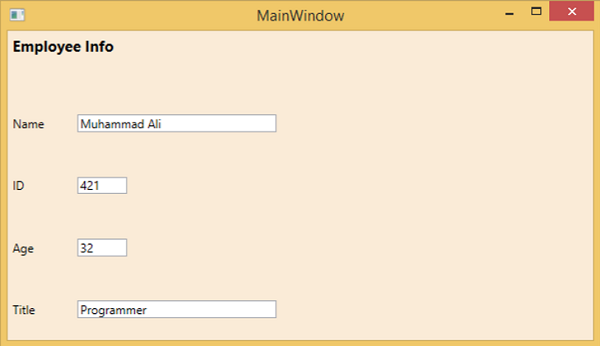# WPF - Nesting of Layout

Nesting of layout means the use layout panel inside another layout, e.g. define stack panels inside a grid. This concept is widely used to take the advantages of multiple layouts in an application. In the following example, we will be using stack panels inside a grid.

Let’s have a look at the following XAML code.

```<Window x:Class = "WPFNestingLayouts.MainWindow"
xmlns = "http://schemas.microsoft.com/winfx/2006/xaml/presentation"
xmlns:x = "http://schemas.microsoft.com/winfx/2006/xaml"
xmlns:d = "http://schemas.microsoft.com/expression/blend/2008"
xmlns:mc = "http://schemas.openxmlformats.org/markup-compatibility/2006"
xmlns:local = "clr-namespace:WPFNestingLayouts"
mc:Ignorable = "d" Title = "MainWindow" Height = "350" Width = "604">

<Grid Background = "AntiqueWhite">
<Grid.RowDefinitions>
<RowDefinition Height = "*" />
<RowDefinition Height = "*" />
<RowDefinition Height = "*" />
<RowDefinition Height = "*" />
<RowDefinition Height = "*" />
</Grid.RowDefinitions>

<Grid.ColumnDefinitions>
<ColumnDefinition Width = "*" />
</Grid.ColumnDefinitions>

<Label Content = "Employee Info" FontSize = "15"
FontWeight = "Bold" Grid.Column = "0" Grid.Row = "0"/>

<StackPanel Grid.Column = "0" Grid.Row = "1" Orientation = "Horizontal">
<Label Content = "Name"  VerticalAlignment = "Center" Width = "70"/>
<TextBox Name = "txtName" Text = "Muhammad Ali" VerticalAlignment = "Center"
Width = "200">
</TextBox>
</StackPanel>

<StackPanel Grid.Column = "0" Grid.Row = "2" Orientation = "Horizontal">
<Label Content = "ID" VerticalAlignment = "Center" Width = "70"/>
<TextBox Name = "txtCity" Text = "421" VerticalAlignment = "Center"
Width = "50">
</TextBox>
</StackPanel>

<StackPanel Grid.Column = "0" Grid.Row = "3" Orientation = "Horizontal">
<Label Content = "Age" VerticalAlignment = "Center" Width = "70"/>
<TextBox Name = "txtState" Text = "32" VerticalAlignment = "Center"
Width = "50"></TextBox>
</StackPanel>

<StackPanel Grid.Column = "0" Grid.Row = "4" Orientation = "Horizontal">
<Label Content = "Title" VerticalAlignment = "Center" Width = "70"/>
<TextBox Name = "txtCountry" Text = "Programmer" VerticalAlignment = "Center"
Width = "200"></TextBox>
</StackPanel>

</Grid>

</Window>
```

When you compile and execute the above code, it will produce the following window.We recommend that you execute the above example code and try other nesting layouts.